# Pi Day Worksheets

#### What is Pi Day?

We all know what Pi is. It is a mathematical constant represented by the lower-case Greek letter π. It is the ratio of a circle's circumference and diameter. It can help us measure angles and understand many different things about circles. The value of Pi is 3.14592654 as per the calculator, and you can also write this as a fraction 22/7, even though it is an irrational number. While we are aware of Pi and its uses, many of us don't know about the 'Pi Day.' It does sound like a festival name, and it indeed is. Taking place every year on March 14th, this day is all about celebrating this mathematical constant 'Pi.' It is celebrated on this specific day because 3, 1, and 4 are the first three significant numbers in this constant.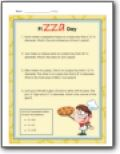###### Pizza Day

A series of word problems related to pizza. Example: Ellen makes two pizzas. One is on a pizza tray that is 12" in diameter. The other is on a pizza tray that is 8" in diameter. What is the total area of Ellen’s two pizzas?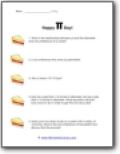###### Happy π Day!

What is the relationship between pi and the diameter and circumference of a circle?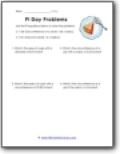###### Circle Problems

A series of problems you must work your way through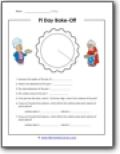###### Bake-Off

This is a live circular problem for you to tackle.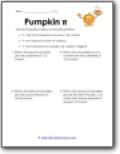###### Pumpkin π

You guessed it! A whole bunch of problems that literally revolve around this sweet treat.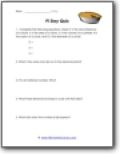###### Quiz

Complete the following equations where C is the circumference of a circle, A is the area of a circle, V is the volume of a cylinder, R is the radius of a circle, and D is the diameter of a circle.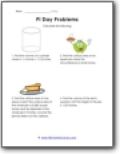###### Pieces of Problems

Example: Find the surface area of one piece of pie if the surface area of the whole pie is 63.585 square inches and the diameter of the whole pie is 9 inches. Assume the pie has been cut into 6 pieces.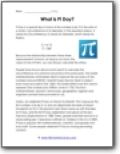###### What is It?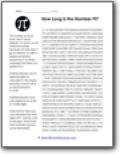###### How Long is the Number?

The number pi never ends, and it never repeats. It is known as an irrational number because not only does it go on forever, it is also a perpetual random sequence of numbers, meaning that there is no pattern to it.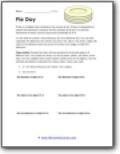###### Circular Objects

Provide the class with an assortment of circular objects of different sizes. You could use circles cut out of paper, plates, pie plates, pizza trays, or even cookies! Have each student choose one or two objects.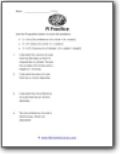###### More Practice

Solve all the problems.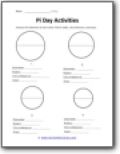###### Activities

Measure the diameter of each circle. Find its radius, circumference, and area.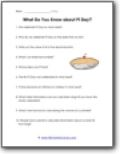###### What Do You Know About It?

What is an irrational number?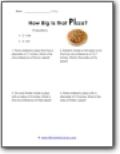###### How Big Is that Pi-zza?

Roberto made a mini pizza crust that has circumference of 15.7 inches. What is the radius of his pizza?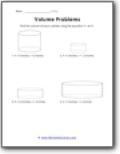###### Volume Problems

Find the volume of each cylinder using the equation.